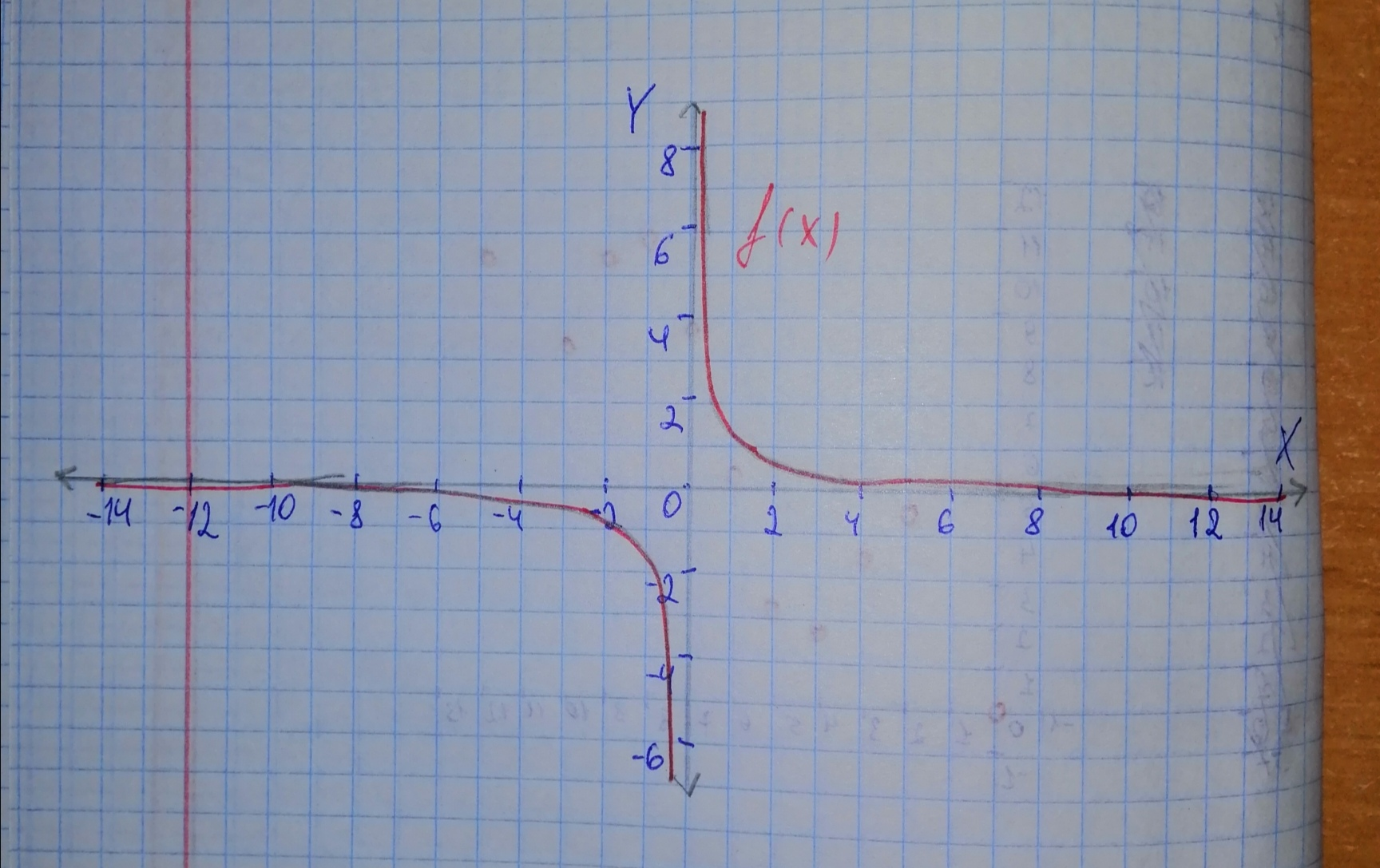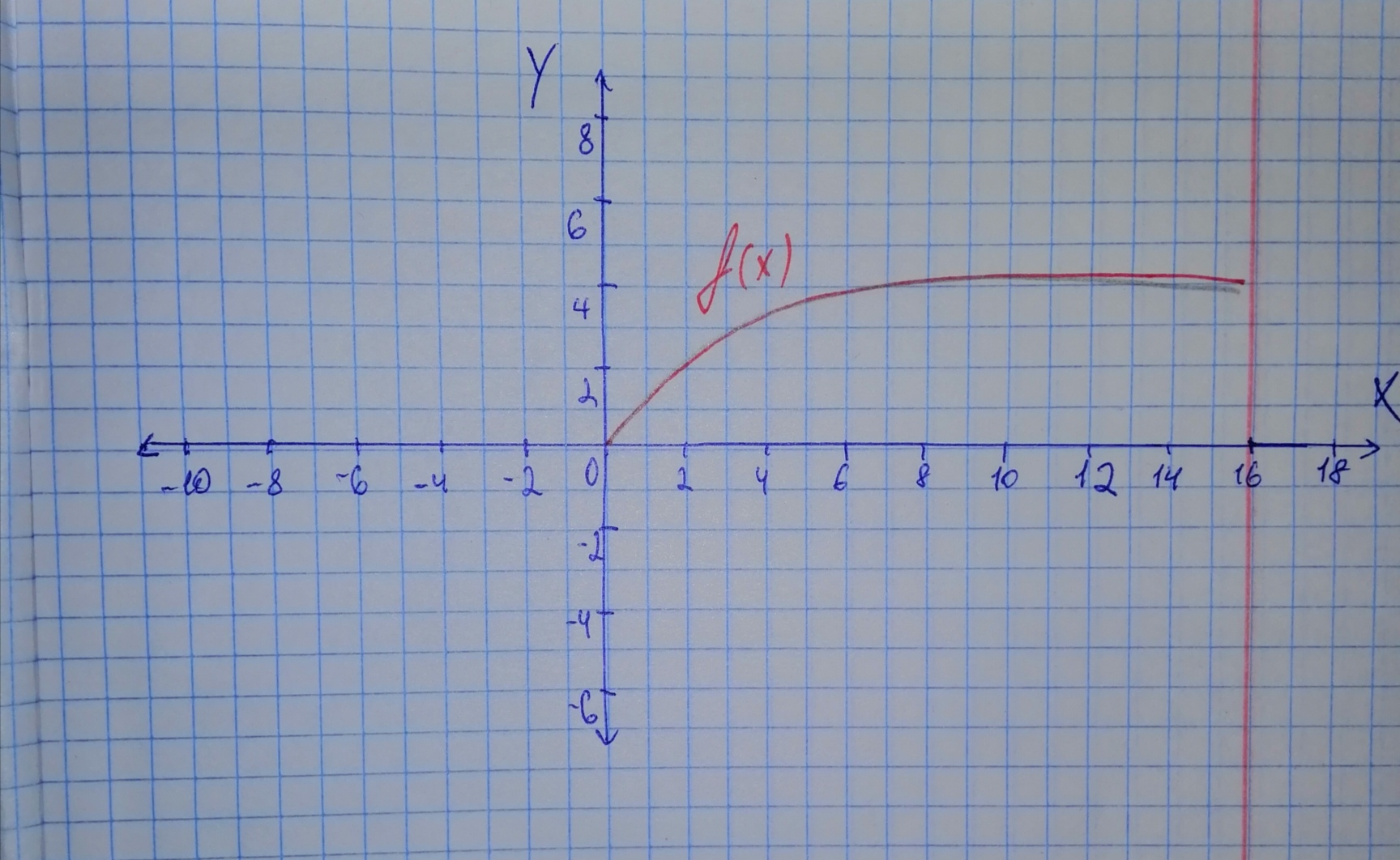tinfoQ

2021-02-25

Sketch the graphs of two functions that are not polynomial functions.
a) $f\left(x\right)=\frac{1}{x}$
b) $f\left(x\right)=\sqrt{x}$Luvottoq

a) The function $f\left(x\right)=\frac{1}{x}$ is not a polynomial because it is not written in the formb) The function $f\left(x\right)=\sqrt{x}$ is not a polynomial because it not written in the formDo you have a similar question?